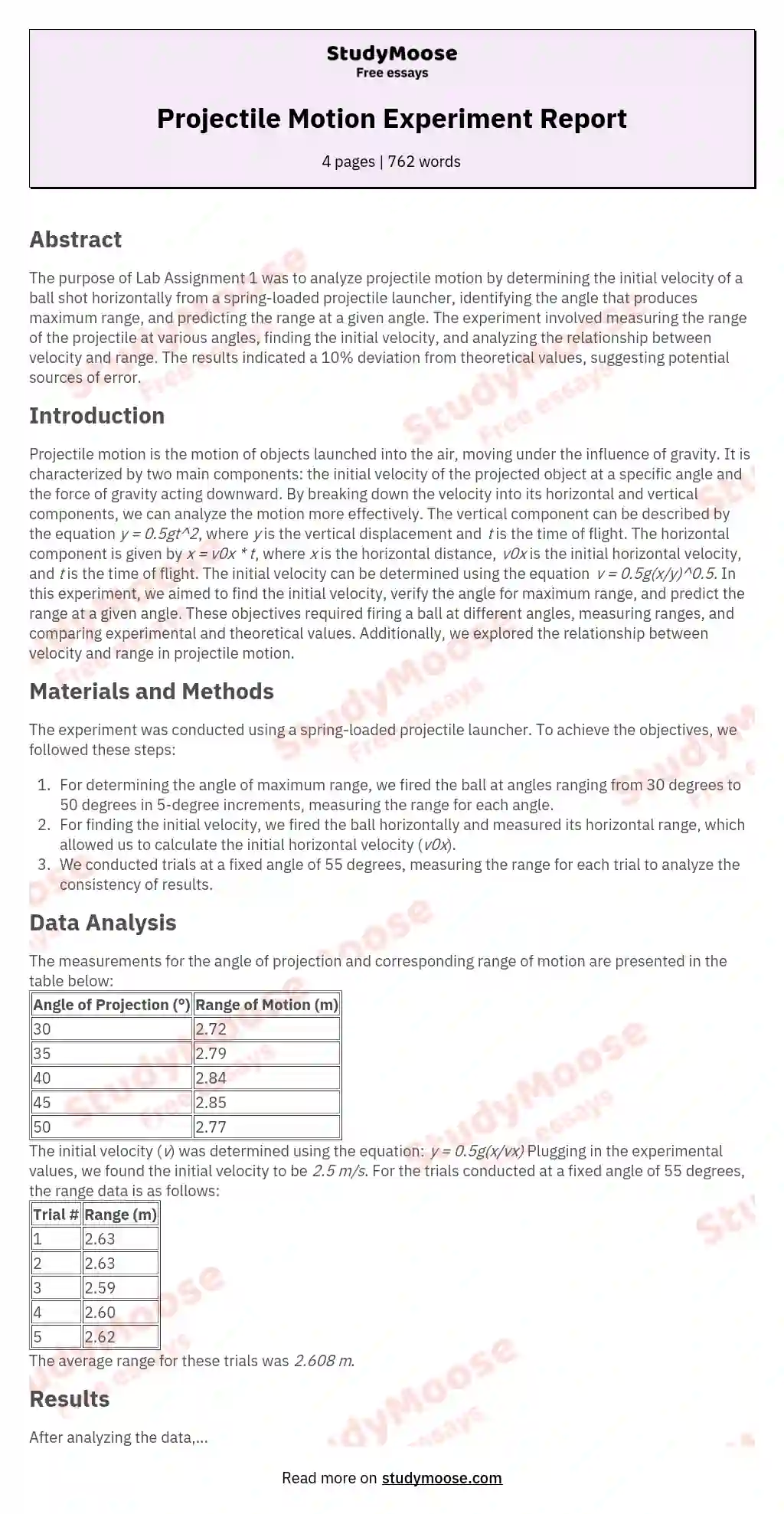# Projectile Motion Lab Report: Lab Assignment 1

Categories: MotionPhysics

The purpose of Lab Assignment 1 was to analyze projectile motion. In doing so, we determined the initial velocity of the ball shot horizontally from the spring loaded projectile launcher. Also, we verified the angle at which the projection of the ball would produce a maximum range. Lastly, we predicted the range that a ball would travel at a certain angle, theta. Projectile motion is the motion of objects that are initially launched, or projected, and then continue moving with only the force of gravity acting upon it.

The forces involved in projectile motion are the initial velocity of the projected object at a certain angle and gravity acting downward on the object. The vector nature of forces can be used to determine how far an object launched can go and its initial velocity at an angle of 0 by finding its x and y components separately. The components of velocity are found by taking the initial velocity multiplied by sin for the y component, and cos for the x component.

Get quality help nowDoctor JenniferVerified writer

Proficient in: Motion5 (893)

“ Thank you so much for accepting my assignment the night before it was due. I look forward to working with you moving forward ”+84 relevant experts are online

To find the initial velocity, we had to plug in specific values into the equation, v=0.5g(x/y), all raised to the one-half power, which was equal to 2.5 m/s.

This equation is derived from the equation for the vertical component of the motion which is y=0.5gty. Also, the time of flight can be found. It can be found with the equation y=y0+v0yt-0.5gt. After solving for t, we find that the ball is in flight for 0.

Get to Know The Price Estimate For Your Paper
Topic
Number of pages
Email Invalid email

You won’t be charged yet!

94 seconds. After finding the time of flight, we were able to then find how far the ball went, or the range, by using the initial velocity and time of flight by the equation x=voxt, to find that the range is equal to 2.35 meters. The procedure to find all the values varied to find each one. First, to find the maximum range at which the ball travels, the ball had to be fired at various angles. After doing so, we found that an angle of 45 produces that greatest range.

Second, to find the initial velocity we fired the ball completely horizontally and measured how far it went and used the initial velocity to find the resulting initial velocity. Lastly, we fired the ball at an angle of 55 five times and found the average range and compared our experimental value with the theoretical value to come up with a 10% error. While determining the angle of maximum range, we used the launcher to shoot the ball at different angles, starting at 30 degrees and increasing by 5 degree increments up to 50 degrees, and then determined the point at which the ball landed for each angle of projection. The measurements are as follows: Angle of Projection ()| Range of motion (m)|

30| 2.72|
35| 2.79|
40| 2.84|
45| 2.85|
50| 2.77|

The initial velocity we found from the experiment was 2.5 m/s. We found this using equation 2 from the lab handbook:
y=0.5g(x/vx)
Plugging in the values from our experiment we get:
0.89=0.5(9.81)(1.85/vx)
Solving for initial velocity we get v = 2.5 m/s.

If you fire the projectile launcher at some angle , you can analyze its horizontal and vertical motion independently of each other. The angle we chose for this part of the experiment was 55 degrees. Our data is as follows:

Trial #| Range (m)|
1| 2.63|
2| 2.63|
3| 2.59|
4| 2.60|
5| 2.62|
Average| 2.608|

After analyzing all of our data from the experiment, we realize that the initial velocity can be used for all trials because the projectile launcher has the same initial velocity each time it was shot for the medium range. Once we found the initial velocity, we could use it to find the range and how long the ball was in the air. Our percent deviation in the range was 10% which is rather high considering the experiment and conditions. In analysis, we see that doubling the velocity causes the range to increase by four times, because the range and initial velocity are directly related (x is directly related to v).

Further, the range will be affected just a little bit (approximately 1.4 times) further when doubling the initial height. In this experiment we learned how to determine the initial velocity of a ball that is launched horizontally out of a projectile launcher, how to verify the angle of projection that will produce maximum range, and to predict and verify the range that a ball will travel when launched at a set angle. In conclusion, the final range we predicted compared to the range we found in the experiment was no good because our percent deviation was 10%. This could be due to many different errors, such as errors in measurement, air resistance, stability, etc.